# 300 mm 300 mm Determine the internal forces at Point J when a=0. lec 480 mm...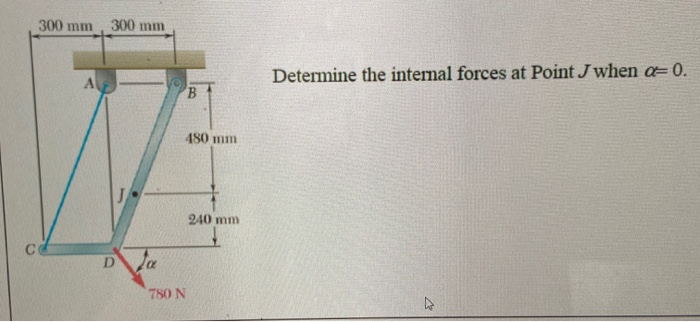300 mm 300 mm Determine the internal forces at Point J when a=0. lec 480 mm 240 mm С D 780 N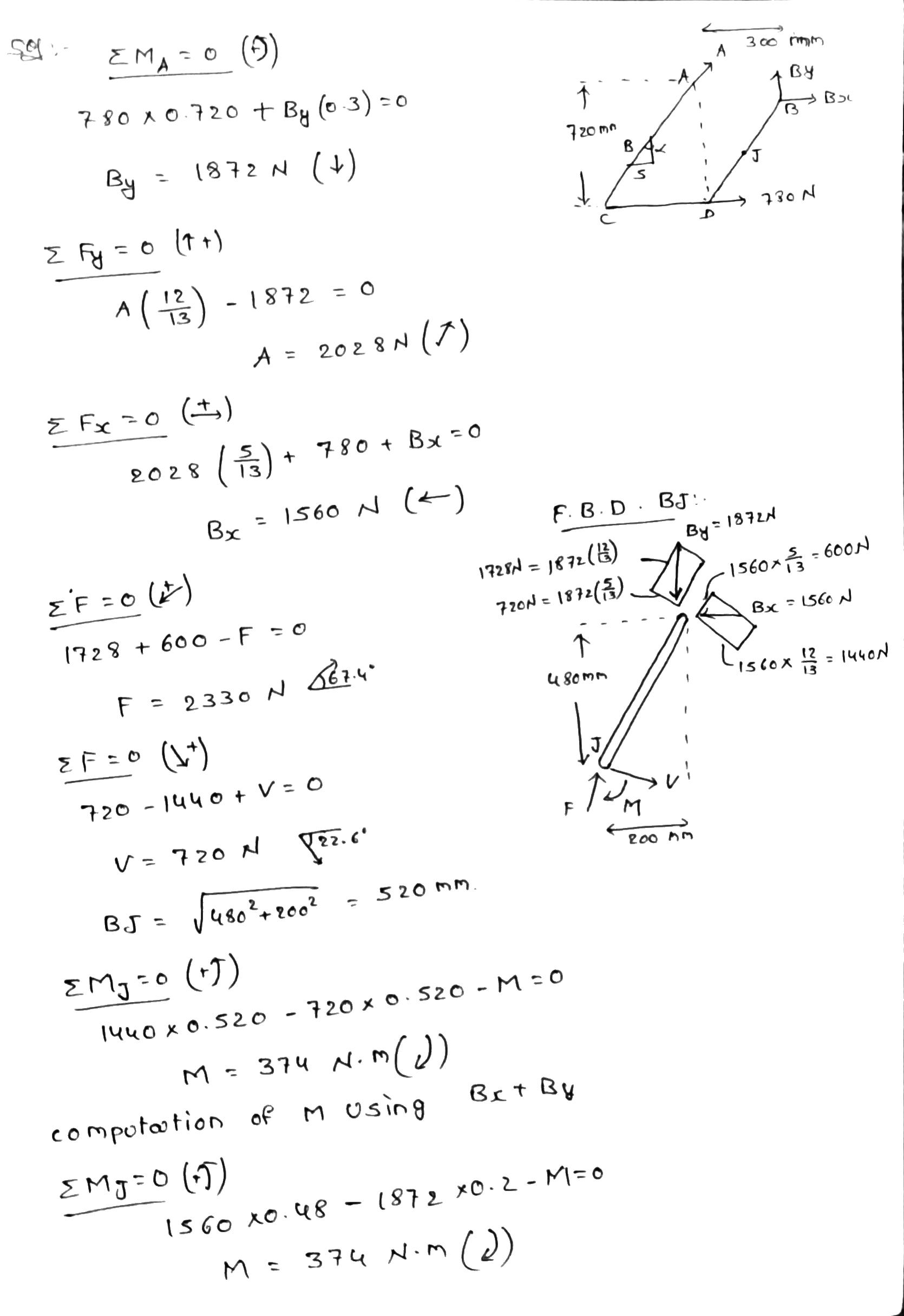##### Add Answer of: 300 mm 300 mm Determine the internal forces at Point J when a=0. lec 480 mm...
Similar Homework Help Questions
• ### 300 mm 300 mm Determine the internal forces at Point J when a=0. A B 480...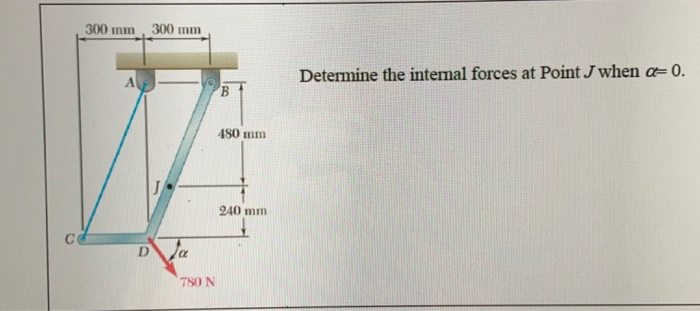300 mm 300 mm Determine the internal forces at Point J when a=0. A B 480 mm 240 mm D 780 N

• ### Determ ine the internal forces at point J for the structure shown, where member BD can...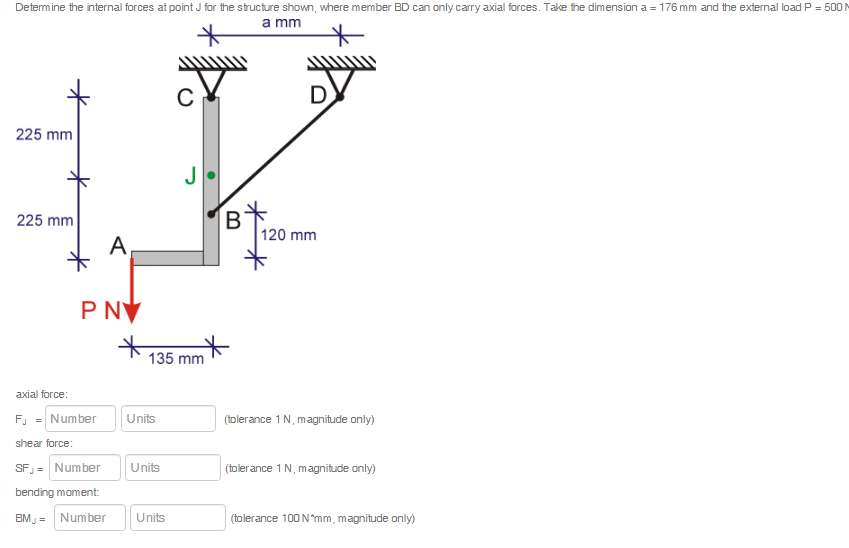Determ ine the internal forces at point J for the structure shown, where member BD can only carry axial forces. Take the dimension a 176 mm and the external load P 500 M a mm 225 mm B 120 mm 225 mm A P NV 135 mm axial force: Number Units (tolerance 1 N, magnitude only) FJ shear force: SF= Number Units (tolerance 1 N, magnitude only) bending moment Number Units (tolerance 100 N mm, magnitude only) BMJ Determ ine...

• ### PROBLEM 4.28 800 mm Determine the reactions at A and C when (a) a-0, 300 N...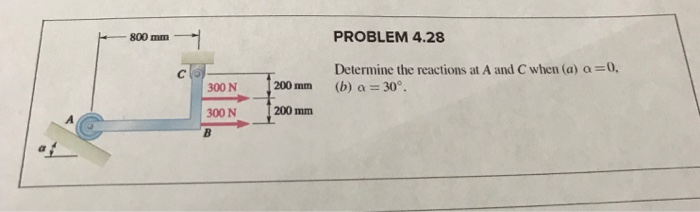PROBLEM 4.28 800 mm Determine the reactions at A and C when (a) a-0, 300 N 200mm (b) a 30 (b) a 30 200 mm 300 N

• ### Internal forcesKnowing that the radius of each pulley us 125 mm and neglectingfrction, determine the internal forces at point K of the frameshow. I managed to find the ractions at D and A but i'm stuck with therest of the problem.Any help would be appreciated.

• ### INFLUENCE LINE - ASSIGNMENT A С B 140 mm 300 mm 140 mm The configuration of...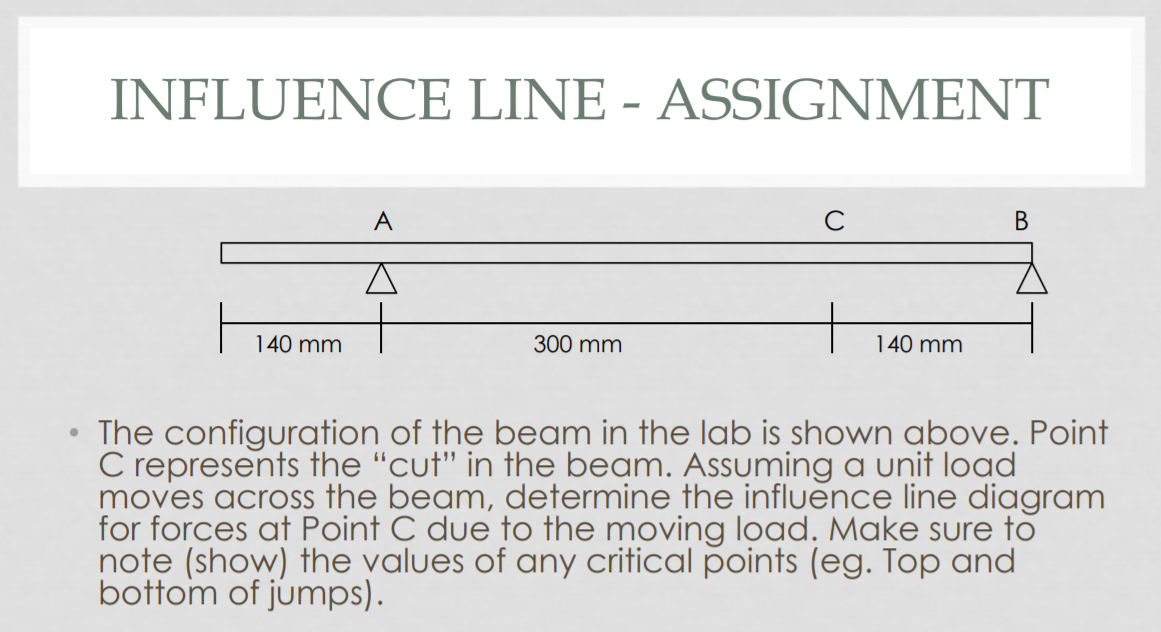INFLUENCE LINE - ASSIGNMENT A С B 140 mm 300 mm 140 mm The configuration of the beam in the lab is shown above. Point C represents the "cut" in the beam. Assuming a unit load moves across the beam, determine the influence line diagram for forces at Point C due to the moving load. Make sure to note (show) the values of any critical points (eg. Top and bottom of jumps).

• ### Knowing that the tension in cable BCis 760 N, determine the resultant of the three forces...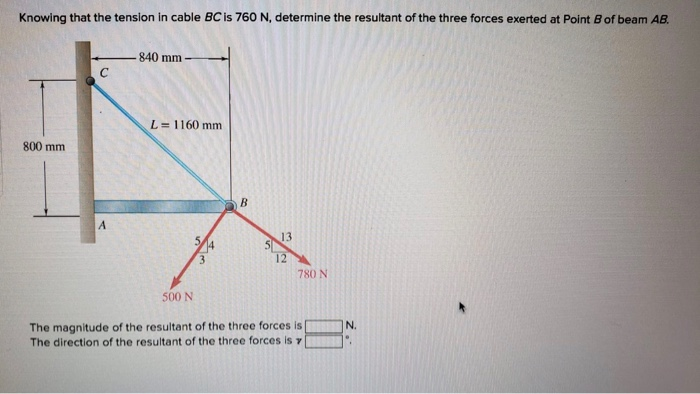Knowing that the tension in cable BCis 760 N, determine the resultant of the three forces exerted at Point B of beam AB 840 mm- L= 1160 mm 800 mm 13 12 780 N 500 N The magnitude of the resultant of the three forces is The direction of the resultant of the three forces is γ N.

• ### Determine ( a ) The moment F = 300 N نقطتان about point o 500 N...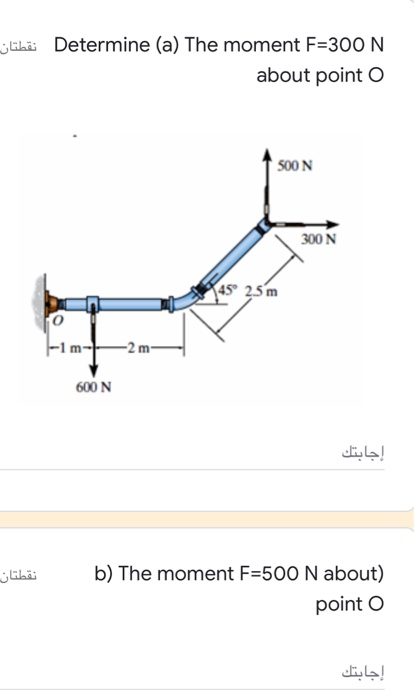Determine ( a ) The moment F = 300 N نقطتان about point o 500 N 300N (45 25m -2 m- 600N إجابتك نقطتان b) The moment F=500 N about) point o إجابتك abä с) The moment F=600 N about point) O إجابتك d) the resultant moment produced) .by the forces about point o إجابتك

• ### 4) Two forces are applied as shown to the rod AB, which is welded to the...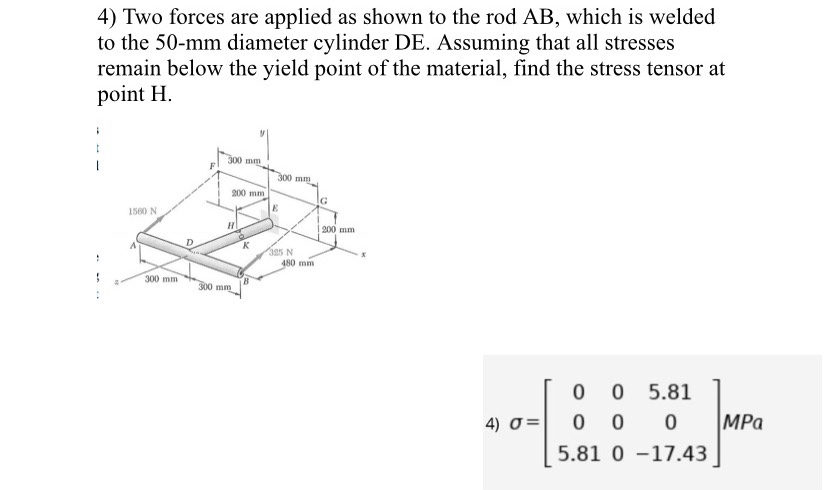4) Two forces are applied as shown to the rod AB, which is welded to the 50-mm diameter cylinder DE. Assuming that all stresses remain below the yield point of the material, find the stress tensor at point H. Answer is below. 4) Two forces are applied as shown to the rod AB, which is welded to the 50-mm diameter cylinder DE. Assuming that all stresses remain below the yield point of the material, find the stress tensor at point...

• ### 300 N-m 500 N-m 3. Consider the torque-loaded member shown. Determine the internal torque in each...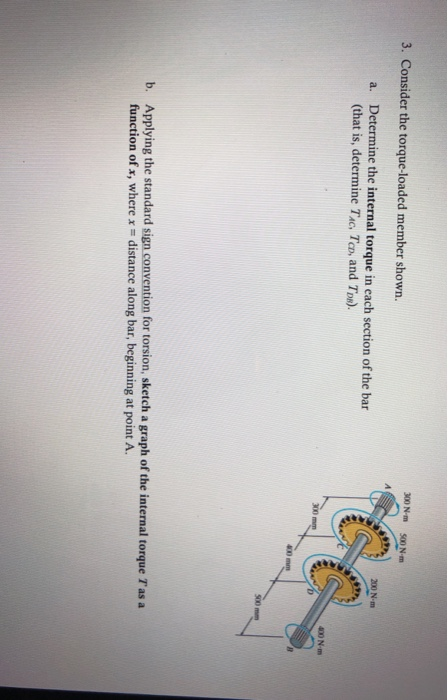300 N-m 500 N-m 3. Consider the torque-loaded member shown. Determine the internal torque in each section of the bar (that is, determine TAc. Tco, and Tos). a. 300 400 mm 500 mm Applying the standard sign convention for torsion, sketch a graph of the internal torque T as a function of x, where b. - distance along bar, beginning at point A.

• ### 6.53 Determine the components of all forces acting on member ABCD when 0 = 0. 4...6.53 Determine the components of all forces acting on member ABCD when 0 = 0. 4 in. 4 in. 2 in. -12 in. E B с D 8 in. F 60 lb o

Free Homework App